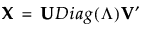Publication date: 06/16/2020

## The Singular Value Decomposition

The singular value decomposition (SVD) enables you to express any linear transformation as a rotation, followed by a scaling, followed by another rotation. The SVD states that any n by p matrix X can be written as follows:Let r be the rank of X. Denote the r by r identity matrix by Ir.

The matrices U, Diag(Λ), and V have the following properties:

U is an n by r semi-orthogonal matrix with UU = Ir

V is a p by r semi-orthogonal matrix with VV = Ir

Diag(Λ) is an r by r diagonal matrix with positive diagonal elements given by the column vector Λ = (λ1, λ2, ..., λr) where λ1 ≥ λ2 ≥ ... ≥ λr > 0.

The λi are the nonzero singular values of X.

The following statements relate the SVD to the spectral decomposition of a square matrix:

The squares of the λi are the nonzero eigenvalues of XX.

The r columns of V are eigenvectors of XX.

Note: There are various conventions in the literature regarding the dimensions of the matrices U, V, and the matrix containing the singular values. However, the differences have no practical impact on the decomposition up to the rank of X.